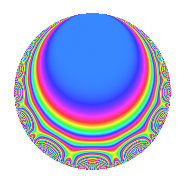# Properties

 Label 2007.1.d.cLevel 2007 Weight 1 Character orbit 2007.d Self dual Yes Analytic conductor 1.002 Analytic rank 0 Dimension 6 Projective image $$D_{14}$$ CM disc. -223 Inner twists 4

# Related objects

## Newspace parameters

 Level: $$N$$ = $$2007 = 3^{2} \cdot 223$$ Weight: $$k$$ = $$1$$ Character orbit: $$[\chi]$$ = 2007.d (of order $$2$$ and degree $$1$$)

## Newform invariants

 Self dual: Yes Analytic conductor: $$1.00162348035$$ Analytic rank: $$0$$ Dimension: $$6$$ Coefficient field: $$\Q(\zeta_{28})^+$$ Coefficient ring: $$\Z[a_1, a_2]$$ Coefficient ring index: $$1$$ Projective image $$D_{14}$$ Projective field Galois closure of $$\mathbb{Q}[x]/(x^{14} - \cdots)$$

## $q$-expansion

Coefficients of the $$q$$-expansion are expressed in terms of a basis $$1,\beta_1,\ldots,\beta_{5}$$ for the coefficient ring described below. We also show the integral $$q$$-expansion of the trace form.

 $$f(q)$$ $$=$$ $$q$$ $$-\beta_{1} q^{2}$$ $$+ ( 1 + \beta_{2} ) q^{4}$$ $$+ ( 1 - \beta_{2} - \beta_{4} ) q^{7}$$ $$+ ( -\beta_{1} - \beta_{3} ) q^{8}$$ $$+O(q^{10})$$ $$q$$ $$-\beta_{1} q^{2}$$ $$+ ( 1 + \beta_{2} ) q^{4}$$ $$+ ( 1 - \beta_{2} - \beta_{4} ) q^{7}$$ $$+ ( -\beta_{1} - \beta_{3} ) q^{8}$$ $$+ ( \beta_{3} + \beta_{5} ) q^{14}$$ $$+ ( 2 \beta_{2} + \beta_{4} ) q^{16}$$ $$-\beta_{5} q^{17}$$ $$-\beta_{2} q^{19}$$ $$+ q^{25}$$ $$+ ( 1 - 2 \beta_{2} - 2 \beta_{4} ) q^{28}$$ $$+ \beta_{1} q^{29}$$ $$+ \beta_{4} q^{31}$$ $$+ ( -\beta_{1} - \beta_{3} - \beta_{5} ) q^{32}$$ $$+ ( -1 + \beta_{2} + 2 \beta_{4} ) q^{34}$$ $$+ ( -1 + \beta_{2} + \beta_{4} ) q^{37}$$ $$+ ( \beta_{1} + \beta_{3} ) q^{38}$$ $$+ \beta_{3} q^{41}$$ $$-\beta_{4} q^{43}$$ $$+ \beta_{5} q^{47}$$ $$+ ( 1 - \beta_{4} ) q^{49}$$ $$-\beta_{1} q^{50}$$ $$+ \beta_{5} q^{53}$$ $$+ ( \beta_{1} + \beta_{3} + \beta_{5} ) q^{56}$$ $$+ ( -2 - \beta_{2} ) q^{58}$$ $$-\beta_{5} q^{62}$$ $$+ ( 2 \beta_{2} + 2 \beta_{4} ) q^{64}$$ $$+ ( -\beta_{3} - \beta_{5} ) q^{68}$$ $$+ \beta_{4} q^{73}$$ $$+ ( -\beta_{3} - \beta_{5} ) q^{74}$$ $$+ ( -1 - 2 \beta_{2} - \beta_{4} ) q^{76}$$ $$+ ( 1 - 2 \beta_{2} - \beta_{4} ) q^{82}$$ $$+ \beta_{1} q^{83}$$ $$+ \beta_{5} q^{86}$$ $$-\beta_{3} q^{89}$$ $$+ ( 1 - \beta_{2} - 2 \beta_{4} ) q^{94}$$ $$+ ( -\beta_{1} + \beta_{5} ) q^{98}$$ $$+O(q^{100})$$ $$\operatorname{Tr}(f)(q)$$ $$=$$ $$6q$$ $$\mathstrut +\mathstrut 8q^{4}$$ $$\mathstrut +\mathstrut 2q^{7}$$ $$\mathstrut +\mathstrut O(q^{10})$$ $$6q$$ $$\mathstrut +\mathstrut 8q^{4}$$ $$\mathstrut +\mathstrut 2q^{7}$$ $$\mathstrut +\mathstrut 6q^{16}$$ $$\mathstrut -\mathstrut 2q^{19}$$ $$\mathstrut +\mathstrut 6q^{25}$$ $$\mathstrut -\mathstrut 2q^{28}$$ $$\mathstrut +\mathstrut 2q^{31}$$ $$\mathstrut -\mathstrut 2q^{37}$$ $$\mathstrut -\mathstrut 2q^{43}$$ $$\mathstrut +\mathstrut 4q^{49}$$ $$\mathstrut -\mathstrut 14q^{58}$$ $$\mathstrut +\mathstrut 8q^{64}$$ $$\mathstrut +\mathstrut 2q^{73}$$ $$\mathstrut -\mathstrut 12q^{76}$$ $$\mathstrut +\mathstrut O(q^{100})$$

Basis of coefficient ring in terms of $$\nu = \zeta_{28} + \zeta_{28}^{-1}$$:

 $$\beta_{0}$$ $$=$$ $$1$$ $$\beta_{1}$$ $$=$$ $$\nu$$ $$\beta_{2}$$ $$=$$ $$\nu^{2} - 2$$ $$\beta_{3}$$ $$=$$ $$\nu^{3} - 3 \nu$$ $$\beta_{4}$$ $$=$$ $$\nu^{4} - 5 \nu^{2} + 5$$ $$\beta_{5}$$ $$=$$ $$\nu^{5} - 5 \nu^{3} + 5 \nu$$
 $$1$$ $$=$$ $$\beta_0$$ $$\nu$$ $$=$$ $$\beta_{1}$$ $$\nu^{2}$$ $$=$$ $$\beta_{2}\mathstrut +\mathstrut$$ $$2$$ $$\nu^{3}$$ $$=$$ $$\beta_{3}\mathstrut +\mathstrut$$ $$3$$ $$\beta_{1}$$ $$\nu^{4}$$ $$=$$ $$\beta_{4}\mathstrut +\mathstrut$$ $$5$$ $$\beta_{2}\mathstrut +\mathstrut$$ $$5$$ $$\nu^{5}$$ $$=$$ $$\beta_{5}\mathstrut +\mathstrut$$ $$5$$ $$\beta_{3}\mathstrut +\mathstrut$$ $$10$$ $$\beta_{1}$$

## Character Values

We give the values of $$\chi$$ on generators for $$\left(\mathbb{Z}/2007\mathbb{Z}\right)^\times$$.

 $$n$$ $$226$$ $$893$$ $$\chi(n)$$ $$-1$$ $$1$$

## Embeddings

For each embedding $$\iota_m$$ of the coefficient field, the values $$\iota_m(a_n)$$ are shown below.

For more information on an embedded modular form you can click on its label.

Label $$\iota_m(\nu)$$ $$a_{2}$$ $$a_{3}$$ $$a_{4}$$ $$a_{5}$$ $$a_{6}$$ $$a_{7}$$ $$a_{8}$$ $$a_{9}$$ $$a_{10}$$
1783.1
 1.94986 1.56366 0.867767 −0.867767 −1.56366 −1.94986
−1.94986 0 2.80194 0 0 −1.24698 −3.51352 0 0
1783.2 −1.56366 0 1.44504 0 0 1.80194 −0.695895 0 0
1783.3 −0.867767 0 −0.246980 0 0 0.445042 1.08209 0 0
1783.4 0.867767 0 −0.246980 0 0 0.445042 −1.08209 0 0
1783.5 1.56366 0 1.44504 0 0 1.80194 0.695895 0 0
1783.6 1.94986 0 2.80194 0 0 −1.24698 3.51352 0 0
 $$n$$: e.g. 2-40 or 990-1000 Embeddings: e.g. 1-3 or 1783.6 Significant digits: Format: Complex embeddings Normalized embeddings Satake parameters Satake angles

## Inner twists

Char. orbit Parity Mult. Self Twist Proved
1.a Even 1 trivial yes
223.b Odd 1 CM by $$\Q(\sqrt{-223})$$ yes
3.b Odd 1 yes
669.c Even 1 yes

## Hecke kernels

This newform can be constructed as the kernel of the linear operator $$T_{2}^{6}$$ $$\mathstrut -\mathstrut 7 T_{2}^{4}$$ $$\mathstrut +\mathstrut 14 T_{2}^{2}$$ $$\mathstrut -\mathstrut 7$$ acting on $$S_{1}^{\mathrm{new}}(2007, [\chi])$$.